• 一维搜索一般有如下方法，解析法和数值解法。解析法即是按照数学公式求导数求极值。但是一般实际问题中，往往不知道损失函数的数学表达式、或者导数比较难求，这种方法一般应用于科学计算。数值类方法有分为两类，...
[笔记整理] 一维搜索
0x00 摘要
0x01 概念
1.1 一维搜索
一维搜索是用来求步长的。
Line Search顾名思义就是沿着一个线寻找下一个x_{k+1}，
一维搜索的理论是建立在整个最优化理论之下的。如果要理解什么一维搜索，就要理解最优化的目标与形式。
最优化目标: min(f(x))，一般而言f(x)是凸的。
在大多数多变量函数的最优化中，迭代格式为：

x

k

+

1

=

x

k

+

α

k

d

k

x

k

是

已

经

搜

索

到

的

点

搜

索

方

向

(

变

量

变

化

的

方

向

)

:

d

k

步

长

因

子

(

变

量

变

化

的

大

小

，

也

被

称

为

学

习

率

)

:

α

k

即

1.

求

取

下

降

方

向

d

k

,

使

d

k

<

0

2.

求

取

步

长

α

,

使

f

(

x

+

α

d

)

<

f

(

x

)

3.

反

复

迭

代

直

至

收

敛

\begin{aligned}&x_{k+1} = x_k + \alpha_kd_k \\\\&x_k 是已经搜索到的点 \\&搜索方向(变量变化的方向): d_k \\&步长因子(变量变化的大小，也被称为学习率): \alpha_k \\&即 \\&1. 求取下降方向 d_k, 使d_k < 0\\&2. 求取步长 \alpha, 使f(x+\alpha d ) < f(x) \\&3. 反复迭代直至收敛 \\\end{aligned}

其中最为关键的就是往哪走的搜索方向 d_k 和走多少的步长因子 alpha_k，如果确定了搜索方向，那么求解步长因子 alpha_k 的问题就是一维优化问题，不妨设：

Φ

(

α

)

=

f

(

x

k

+

α

k

d

k

)

则

从

x

k

出

发

，

沿

搜

索

方

向

d

k

，

确

定

步

长

因

子

α

k

，

使

得

Φ

(

α

)

<

Φ

(

0

)

的

问

题

就

是

关

于

步

长

因

子

α

的

一

维

搜

索

问

题

\begin{aligned}&\Phi(\alpha) = f(x_k + \alpha_kd_k ) \\ \\&则从x_k出发，沿搜索方向d_k，确定步长因子 \alpha_k，使得\Phi(\alpha) < \Phi(0)的问题就是关于步长因子 \alpha 的一维搜索问题\end{aligned}

所谓一维搜索指的就是步骤2 “求取步长”。
为了进行一维搜索，我们首先需要缩小变量所在的变化范围，然后再用其他方法去求极值。
一维搜索主要结构可作如下概括：
首先确定包含问题最优解的搜索区间。然后采用某种分割技术或者插值方法缩小这个区间，进行搜索求解。
一维搜索一般有如下方法，解析法和数值解法。解析法即是按照数学公式求导数求极值。但是一般实际问题中，往往不知道损失函数的数学表达式、或者导数比较难求，这种方法一般应用于科学计算。数值类方法有分为两类，试探法和插值法。
一维搜索分为两种：
非精确一维搜索，就是找到一个 alpha 满足一定条件即可，好比Wolfe-Powell,Armijo条件。精确一维搜索，就是找到一个参数 alpha，使得min(f(x))最小，有插值法，黄金分割法，直接法等等。
1.2 不精确一维搜索
精确一维搜索往往需要花费很大的时间。
当迭代点远离问题的解时，精确求解通常不十分有效。很多最优化方法，如牛顿法和拟牛顿法，其收敛速度并不依赖于精确一维搜索过程。  只要保证目标函数有满意的下降，可大大节省计算量
所以实际计算中，一般做不到精确的一维搜索，实际上也没有必要做到这一点，因为精确的一维搜索需要付出较高的代价，对加速收敛作用不大，因此花费计算量较少的不精确的一维搜索技术方法受到更为广泛的重视和欢迎。
确定步长最简单的方法，就是挨个试。从0开始，每隔一定距离计算一下目标函数的值，找出其中最小的那个，对应的步长就是我们要的结果。
显然，这种方法太过暴力，试的次数太多，很影响效率。所以有一些原则可以帮助我们提高效率。
一般而言主要有以下两种准则：Armijo-Goldstein准则 和 Wolf-Powell 准则。
1.2.1 Armijo-Goldstein准则
Armijo-Goldstein准则核心思想就两点：

目标函数值应该有足够的下降一维搜索的步长 lambda 不应该太小

Armijo 又被称为充分下降条件（sufficient decrease condition），又称Armijo condition。

f

(

x

k

+

α

p

k

)

<

=

f

(

x

k

)

+

c

.

α

▽

f

k

T

p

k

0

<

c

<

1

2

▽

f

k

T

p

k

<

0

，

p

k

为

函

数

下

降

方

向

\begin{aligned}&f(x_k + \alpha p_k) <= f(x_k) + c . \alpha ▽ f^{T}_kp_k \\ \\&0 < c < \frac{1}{2} \\&▽f^{T}_kp_k < 0，p_k为函数下降方向\end{aligned}

Armijo condition这个约束太简单了，以至于任意小的步长 alpha 都可以满足该条件。为了保证每次迭代有充分的下降，我们加上Goldstein conditions。

f

(

x

k

)

+

(

1

−

c

)

α

▽

f

k

T

p

k

<

=

f

(

x

k

+

α

p

k

)

<

=

f

(

x

k

)

+

c

.

α

▽

f

k

T

p

k

0

<

c

<

1

2

▽

f

k

T

p

k

<

0

，

p

k

为

函

数

下

降

方

向

\begin{aligned}&f(x_k)+(1-c)\alpha▽f^{T}_kp_k <= f(x_k + \alpha p_k) <= f(x_k) + c . \alpha ▽ f^{T}_kp_k \\ \\&0 < c < \frac{1}{2} \\&▽f^{T}_kp_k < 0，p_k为函数下降方向\end{aligned}

这是两个不等式，左右分别对应斜率不同的直线，将可接受区域约束在这两条直线之间。
如果步长 alpha 太小的话，会导致左边不等式接近于不成立的边缘。因此，左边不等式 就保证了 alpha 不能太小。
Armijo-Goldstein准则有可能把最优步长因子排除在可接受区间外，所以Wolfe-Powell更可以满足我们需求。
1.2.2 Wolf-Powell 准则
Wolfe conditions实际上规定了两件事情，

函数值必须下降只接受导数较平缓的区域

Wolfe conditions使得目标点处的切线变得“不那么斜”了，这符合实际规律，因为极值点处不应该过于陡峭。
既然和导数大小有关，那么我们可以试着考虑二分点的导数值。如果二分点的导数值很小，直接满足Wolfe conditions，那就找到了可接受的步长。如果二分点的导数值很大，那么我们就选择与该点导数符号相反的那个端点，重新组成一个子区间。之所以选择符号相反的那个端点，是因为对于连续光滑的函数来说，两个斜率相反的点之间一定存在极值点，也就存在导数值很小的一段区域。按照这种策略，逐步缩小区间范围，直到某个二分点满足Wolfe conditions为止。
Wolfe-Powell方法相较于精确一维搜索，不再采用进退法去寻找搜索区间。在进退法里面，是通过慢慢扩展生成区间，然后在在区间中查找合适的，而在Wolfe-Powell中我们可以直接定义步长区间的界限，比如[0,10000]，那么其会根据其准则去每次剔除不符合的区间，逐步缩小区间，其能够较为快速的跳过无用的计算，速度要快很多。
Wolfe-Powell方法计算过程中，也用到了插值方法，用以区间切割剔除。
1.2.3 结合代码分析
Armijo准则：首先给一个较大的学习率，然后不断缩减学习率，如果对于函数f(xk+αdk)当前的学习率使函数从当前的位置f(xk)的减小一定程度后还大于预设的期望值，那这个学习率就符合要求了。
什么意思？你看，对于函数f(xk+αdk)，既然是求最小值，那对于当前的值f(xk)减小一定程度后就有个新值f(xk+1)，于是，如果我们将f(xk+1)作为预设的期望值，即我们希望f(xk)在某个学习率α的情况下减小一定程度后可以到达f(xk+1)，那这个α就是我们希望得到的α了对吧。
这个减小程度在Armijo准则中是公式：
c1α▽f(xk)Tdk，c1∈(0, 1)

因为dk一般取梯度负方向，所以用式子表达上面的话的话就是：
f(xk+1)= f(xk) + c1α▽f(xk)Tdk，c1∈(0, 1)

具体分析代码
function lamda = ArmijoGoldstein(func,gfunc,lamda0,ro,apha,iterNum,plotFlag)
if nargin<7
plotFlag = 1;
end
if nargin<6
iterNum = 100;
end
if nargin<5
apha = 2;
end
if nargin<4
ro = 0.1;
end
if nargin<3
lamda0 = 1;
end

a = 0; %左边界
b = inf; %右边界
lamda =lamda0;
f0 = func(0);
g0 = gfunc(0);

while iterNum
flamda = func(lamda);
if flamda<=f0+ro*lamda*g0 %判断Armijo准则
%满足充分下降条件
%if flamda>=f0+(1-ro)*lamda*g0 %代码可以调整为判断Goldstein
if gfunc(lamda)>=sigma*g0 %判断曲率条件
%满足wolf-powell,步长不会太小
break; %找到了可接受的步长，返回即可
else
%不满足wolf-powell，说明当前步长落在了斜率较大的区域，此时有两种可能
a = lamda; %左端的a设置为lamda
if isinf(b)
%如果是远离初始点的区域，那么在初始点和当前点之间一定存在可接受的区域。右端的b为空的时候，说明Armijo准则一直是满足的，步长太小了，扩大步长
lamda = alpha*lamda;
else
%如果是接近初始点的区域，这一区域不是我们想要的，需要进一步放大步长
lamda = (a+b)/2; %步长设定为区间的中值
end
end
else
%找到了不满足充分下降条件的步长，因此该步长可以作为右边界，缩小b和步长
b = lamda; %收缩
lamda = (a+b)/2;
end
iterNum = iterNum - 1;
end

%example
%lamda = WolfPowell(@(x)(sin(-4+x)-1),@(x)(cos(-4+x)),1,0.4,0.45,2,100,1)

0xFF 参考
优化方法基础系列-精确的一维搜索技术
优化方法基础系列-非精确的一维搜索技术
[原创]用“人话”解释不精确线搜索中的Armijo-Goldstein准则及Wolfe-Powell准则
https://www.zhihu.com/question/36425542
一维搜索算法介绍及其实现
数值优化|笔记整理（1）——引入，线搜索：步长选取条件
https://zhuanlan.zhihu.com/p/32821110
线搜索（一）：步长的选取
最优化问题——一维搜索(二)
展开全文机器学习
• 一维搜索算法介绍及其实现 当进行优化算法实现时、一般都要进行一系列如下格式的迭代运算 x(k+1) = x(k) + a(k) *s(k)，其中s(k)是变量变化的方向，而a(k)可以确定变量...一维搜索一般有如下方法，解析法和数值...
一维搜索算法介绍及其实现
当进行优化算法实现时、一般都要进行一系列如下格式的迭代运算
x(k+1) = x(k) + a(k) *s(k)，其中s(k)是变量变化的方向，而a(k)可以确定变量变化的大小，即我们平时所说的步长，a(k)被称为学习率。求最佳步长即求一元函数: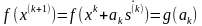的极值问题，这一过程被称为一维搜索。一维搜索也是解多维最优化问题的重要支柱。
一维搜索一般有如下方法，解析法和数值解法。解析法即是按照数学公式求导数求极值。但是一般实际问题中，往往不知道损失函数的数学表达式、或者导数比较难求，这种方法一般应用于科学计算。数值类方法有分为两类，试探法和插值法。
二、确定初始单谷区间的进退法
为了进行一维搜索，我们首先需要缩小变量所在的变化范围，然后再用其他方法去求极值。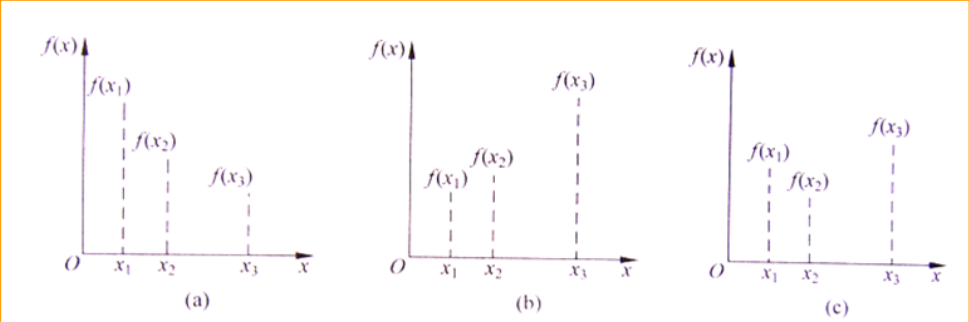如图所示，对于单谷函数而言，有如下结论
若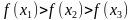，则极小点位于点x2的右边区间。
若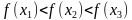，则极小点位于点x2的左边区间。
若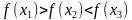，则极小点位于点x1和点x2之间的区间。
x1和x3就是包含一个极小点的区间。
这几个结论告诉我们，只有我们能够找到三点x1,x2,x3使得三点满足条件
x1<x2<x3，f(x1)<f(x2)>f(x3)那么极小值点就肯定在区间[x1,x3]中。
进退法的步骤
1、选定初始点x1,初始步长h，x2 = x1 + h，计算y1 = f(x1),y2 = f(x2)
2、比较y1 和y2
（a）y1 > y2，那么我们已经找到了符合条件的x1和x2，可以继续下一步.
（b）y1 < y2，那么我们找错了方向，应该反向搜搜，将x1 与x2互换，并将h= -h
（c）y1 = y2 ，终止程序，极小值点在区间[x1,x2]之间。
3、产生新的探测点x3 = x2 +h ,y3 = f(x3)
4、比较y2和y3
（a）y2 > y3 ，那么赋值x1 = x2, x2 = x3，转步骤3 继续进行探测。
（b）y2< y3，那么我们已经找到三个符合条件的点了。
极小值所在的区间为[min(x1,x3), max(x1,x3)]

三、区间消退法
搜索区间确定后，在搜索区间[a,b]内任取两点a1 b1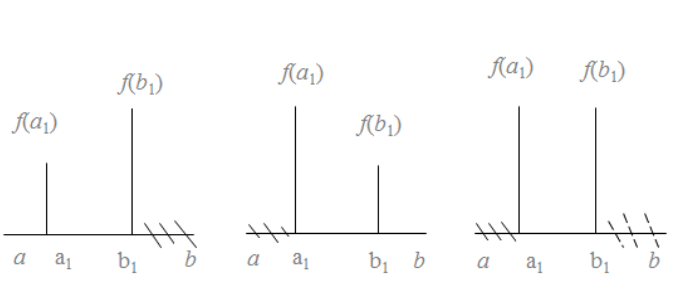若f(a1) < f(b1)，则取[a, b1]为缩短后的搜索区间,反之则取[a1, b]为搜索后的区间，如此迭代下去，当精度满足时，则停止迭代。

四、黄金分割法
黄金分割法也是逐步缩小区间，不过它是按照一定比例进行缩小，步骤如下。
在搜索区间[a,b]，若精度不满足要求，则下次的搜索区间为
a1 = a + 0.382(b-a)
b1 = a + 0.618(b-a)
当精度满足时，则停止迭代。
代码参考地址https://github.com/delltower/machine_learn
展开全文• 一维搜索-黄金分割法matlab实现前言1、黄金分割法1.1 黄金分割法的定义1.2 黄金分割法的搜索过程2、黄金分割matlab实现2.1 求f(x)=x^2-7*x+10的极值2.2 解析法验算：3.黄金分割脚本编码4.附上powell法链接 前言 ...


一维搜索-黄金分割法matlab实现
前言1、黄金分割法1.1 黄金分割法的定义1.2 黄金分割法的搜索过程
2、黄金分割matlab实现2.1 求f(x)=x^2-7*x+10的极值2.2 解析法验算：
3.黄金分割脚本编码4.附上powell法链接

前言
求解优化问题可以用解析解法和数值解法,在很多情况下,机械优化设计问题限制条件比较多,与之对应的数学描述也比较复杂,不便于甚至不可能用解析法求解,只能用数值法求解,如黄金分割法、消去法、牛顿法、二次插值法、共轭梯度法等。本文介绍的是黄金分割法

提示：以下是本篇文章正文内容，下面案例可供参考
1、黄金分割法
1.1 黄金分割法的定义
黄金分割法,又称作0.618法,它是用于一元函数 f (x) 在给定的区间[a b]内搜索极小值点的一种一维搜索方法。“黄金分割”是指将一线段分成两段,使整段长与较长段的长度比值等于较长段与较短段长度的比值,即1:L=L:(1-L),解得:L=0.618.
1.2 黄金分割法的搜索过程
黄金分割法要求插入点 x1 、x2 的位置相对于区间 [a b]两端点具有对称性,即在给定区间 [a b]内取点.
x1=b-L(b-a)x2=a+L(b-a) 如果f(x1)>f(x2).令a=x1; 如果f(x1)<f(x2)令b=x2,重新开始搜索。具体搜索过程见程序框图。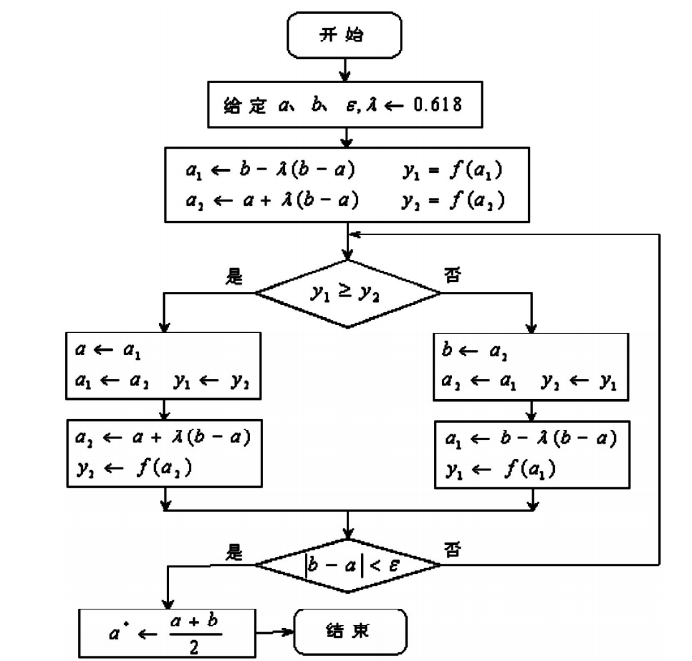2、黄金分割matlab实现
2.1 求f(x)=x^2-7*x+10的极值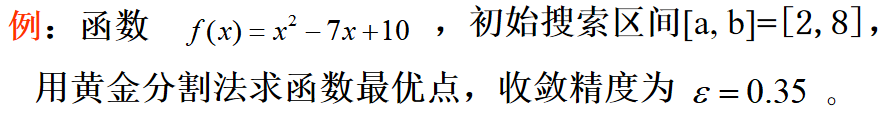代码如下（示例）：
function HJ
syms  x
f=@(x)x^2-7*x+10;
a=2;
b=8;
eps=0.35;
l=0.618;
x1=b-l*(b-a);
x2=a+l*(b-a);
y1=f(x1);
y2=f(x2);
i=0;
while abs(b-a)>eps
i=i+1;

if y1>=y2
a=x1;
x1=x2;
y1=y2;
x2=a+l*(b-a);
y2=f(x2);
else
b=x2;
x2=x1;
y2=y1;
x1=b-l*(b-a);
y1=f(x1);
end
end
x=(a+b)/2
y=f(x)


所得结果为：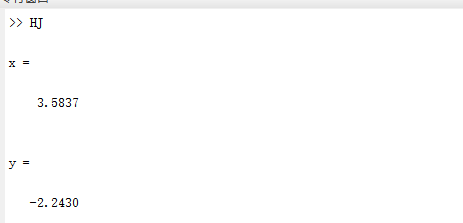2.2 解析法验算：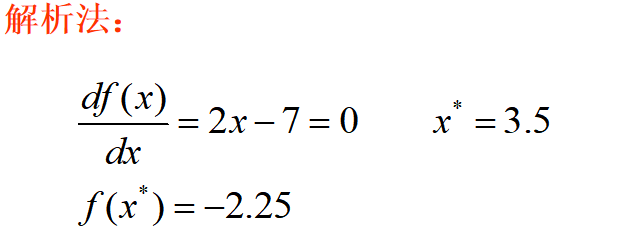3.黄金分割脚本编码
note:此脚本接上文powell法用
function opt_step=goldsection(x01,x02,d,h0)
l=0.618;
[a,b]=search2(x01,x02,d,h0);
a1=b-l*(b-a);y1=ff(x01+d(1)*a1,x02+d(2)*a1);
a2=a+l*(b-a);y2=ff(x01+d(1)*a2,x02+d(2)*a2);
for n=1:100
if y1>=y2
x1(n)=a1;x2(n)=a2;yp1(n)=y1;yp2(n)=y2;
a=a1;a1=a2;y1=y2;
a2=a+l*(b-a);y2=ff(x01+d(1)*a2,x02+d(2)*a2);
else
x1(n)=a1;x2(n)=a2;yp1(n)=y1;yp2(n)=y2;
b=a2;a2=a1;y2=y1;
a1=b-l*(b-a);y1=ff(x01+d(1)*a1,x02+d(2)*a1);
end

aa(n)=(a+b)/2;
y(n)=ff(x01+d(1)*aa(n),x02+d(2)*aa(n));
e(n)=abs(b-a);
opt_step=(a+b)/2;
if abs(b-a)<1e-5
break;
end
end


4.附上powell法链接
powell法
展开全文算法
• 线性方程组 6 种数值解法的对比研究 Gauss消去法、LU分解法、Jacobi迭代法、Gauss-Seidel迭代法、超松弛(SOR)迭代法及共轭迭代法的源程序； 通过实际计算，进一步了解各种方法的优缺点，选择合适的数值方法。 分别...
线性方程组数值解法实验研究
一、实验目的
熟悉求解线性方程组的有关理论和方法；会编写Gauss消去法、LU分解法、Jacobi迭代法、Gauss-Seidel迭代法、超松弛(SOR)迭代法及共轭梯度法的程序；通过实际计算，进一步了解各种方法的优缺点，选择合适的数值方法。
二、实验内容
编程实现Gauss消去法、LU分解法，并用这两种方法求解线性方程组：

⎧⎩⎨⎪⎪x1+2x2−2x3=7x1+x2+x3=22x1+2x2+x3=5(1)

\left\{ \begin{array}{l}
{x_1} + 2{x_2} - 2{x_3} = 7\\
{x_1} + {x_2} + {x_3} = 2\\
2{x_1} + 2{x_2} + {x_3} = 5
\end{array} \right. \tag 1编写Jacobi迭代法、高斯-赛德尔迭代法程序，并用其求解以下84阶线性方程组，并指出各迭代法是否收敛？实验结果说明什么问题？

⎛⎝⎜⎜⎜⎜⎜⎜⎜⎜⎜⎜⎜⎜6816816⋱1⋱8⋱6816816⎞⎠⎟⎟⎟⎟⎟⎟⎟⎟⎟⎟⎟⎟⎛⎝⎜⎜⎜⎜⎜⎜⎜⎜⎜⎜⎜⎜x1x2x3⋮x82x83x84⎞⎠⎟⎟⎟⎟⎟⎟⎟⎟⎟⎟⎟⎟=⎛⎝⎜⎜⎜⎜⎜⎜⎜⎜⎜⎜⎜⎜71515⋮151514⎞⎠⎟⎟⎟⎟⎟⎟⎟⎟⎟⎟⎟⎟(2)

\left( {\begin{array}{*{20}{c}}
6&1&{}&{}&{}&{}&{}\\
8&6&1&{}&{}&{}&{}\\
{}&8&6&1&{}&{}&{}\\
{}&{}& \ddots & \ddots & \ddots &{}&{}\\
{}&{}&{}&8&6&1&{}\\
{}&{}&{}&{}&8&6&1\\
{}&{}&{}&{}&{}&8&6
\end{array}} \right)\left( {\begin{array}{*{20}{c}}
{{x_1}}\\
{{x_2}}\\
{{x_3}}\\
\vdots \\
{{x_{82}}}\\
{{x_{83}}}\\
{{x_{84}}}
\end{array}} \right) = \left( {\begin{array}{*{20}{c}}
7\\
{15}\\
{15}\\
\vdots \\
{15}\\
{15}\\
{14}
\end{array}} \right)\tag 2编写超松弛(SOR)迭代法程序，并用其求解以下4阶方程组，并分析超松弛因子 对SOR收敛性的影响。实验结果说明了什么问题？

⎛⎝⎜⎜⎜13.422−13.42200012.252−12.25200012.377−12.37700011.797⎞⎠⎟⎟⎟⎛⎝⎜⎜⎜x1x2x3x4⎞⎠⎟⎟⎟=⎛⎝⎜⎜⎜750.530010230⎞⎠⎟⎟⎟(3)

\left( {\begin{array}{*{20}{c}}
{13.422}&0&0&0\\
{ - 13.422}&{12.252}&0&0\\
0&{ - 12.252}&{12.377}&0\\
0&0&{ - 12.377}&{11.797}
\end{array}} \right)\left( {\begin{array}{*{20}{c}}
{{x_1}}\\
{{x_2}}\\
{{x_3}}\\
{{x_4}}
\end{array}} \right) = \left( {\begin{array}{*{20}{c}}
{750.5}\\
{300}\\
{102}\\
{30}
\end{array}} \right)\tag 3编写共轭梯度法程序 ，并用其求解问题(1)(2)(3)中的方程组，并求出共轭梯度法在这三种问题下的收敛速度，列出详细的分析过程。
三、实验结果分析
1. Gauss消去法与LU分解法求解线性方程组
根据线性方程组公式(1)可得，系数矩阵

A
$A$ 和常数向量 b$b$

A=⎛⎝⎜112212−211⎞⎠⎟,b=⎛⎝⎜725⎞⎠⎟

A = \left( {\begin{array}{*{20}{c}}
1&2&{ - 2}\\
1&1&1\\
2&2&1
\end{array}} \right),b = \left( {\begin{array}{*{20}{c}}
7\\
2\\
5
\end{array}} \right)
假设

x=[x1x2x3]T

x = {\left[ {\begin{array}{*{20}{c}}
{{x_1}}&{{x_2}}&{{x_3}}
\end{array}} \right]^{\rm{T}}}
由此可得

Ax=b

Ax = b
其中，根据矩阵

A
$A$ 的初等行变换，可以得到矩阵 A$A$ 的秩为3，因此，该线性方程组具有唯一确定解。Gauss消去法和LU分解法都可以得到解。其结果如表1所示。

表1 实验1的结果
方法

x
$x$
计算误差(>2.22E−16$>2.22E-16$)Gauss消去法

[12−1]T
${\left[ {\begin{array}{*{20}{c}}1&2&{{\rm{ - }}1}\end{array}} \right]^{\rm{T}}}$0LU分解法

[12−1]T
${\left[ {\begin{array}{*{20}{c}}1&2&{{\rm{ - }}1}\end{array}} \right]^{\rm{T}}}$0
2. Jacobi迭代法与Gauss-Seidel迭代法求解84阶线性方程组
根据公式(2)，建立线性方程组标准方程

Ax=b

Ax = b
式中

A=⎛⎝⎜⎜⎜⎜⎜⎜⎜⎜⎜⎜⎜⎜6816816⋱1⋱8⋱6816816⎞⎠⎟⎟⎟⎟⎟⎟⎟⎟⎟⎟⎟⎟,b=⎛⎝⎜⎜⎜⎜⎜⎜⎜⎜⎜⎜⎜⎜71515⋮151514⎞⎠⎟⎟⎟⎟⎟⎟⎟⎟⎟⎟⎟⎟

A = \left( {\begin{array}{*{20}{c}}
6&1&{}&{}&{}&{}&{}\\
8&6&1&{}&{}&{}&{}\\
{}&8&6&1&{}&{}&{}\\
{}&{}& \ddots & \ddots & \ddots &{}&{}\\
{}&{}&{}&8&6&1&{}\\
{}&{}&{}&{}&8&6&1\\
{}&{}&{}&{}&{}&8&6
\end{array}} \right),b = \left( {\begin{array}{*{20}{c}}
7\\
{15}\\
{15}\\
\vdots \\
{15}\\
{15}\\
{14}
\end{array}} \right)

x=(x1x2x3⋯x82x83x84)T

x = {\left( {\begin{array}{*{20}{c}}
{{x_1}}&{{x_2}}&{{x_3}}& \cdots &{{x_{82}}}&{{x_{83}}}&{{x_{84}}}
\end{array}} \right)^{\rm{T}}}
基于迭代公式

x(k+1)=Gx(k)+f(4)

{x^{\left( {k + 1} \right)}} = G{x^{\left( k \right)}} + f\tag 4
将系数矩阵

A
$A$ 分解成对角矩阵和上(下)三角矩阵的组合，即
A=D−L−UA = D - L - U
其中

D=diag(666⋯666)

D = {\rm{diag}}\left( {\begin{array}{*{20}{c}}
6&6&6& \cdots &6&6&6
\end{array}} \right)

L=−⎛⎝⎜⎜⎜⎜⎜⎜⎜⎜⎜⎜⎜⎜08080⋱⋱808080⎞⎠⎟⎟⎟⎟⎟⎟⎟⎟⎟⎟⎟⎟

L =  - \left( {\begin{array}{*{20}{c}}
0&{}&{}&{}&{}&{}&{}\\
8&0&{}&{}&{}&{}&{}\\
{}&8&0&{}&{}&{}&{}\\
{}&{}& \ddots & \ddots &{}&{}&{}\\
{}&{}&{}&8&0&{}&{}\\
{}&{}&{}&{}&8&0&{}\\
{}&{}&{}&{}&{}&8&0
\end{array}} \right)

U=−⎛⎝⎜⎜⎜⎜⎜⎜⎜⎜⎜⎜⎜⎜010101⋱⋱01010⎞⎠⎟⎟⎟⎟⎟⎟⎟⎟⎟⎟⎟⎟

U =  - \left( {\begin{array}{*{20}{c}}
0&1&{}&{}&{}&{}&{}\\
{}&0&1&{}&{}&{}&{}\\
{}&{}&0&1&{}&{}&{}\\
{}&{}&{}& \ddots & \ddots &{}&{}\\
{}&{}&{}&{}&0&1&{}\\
{}&{}&{}&{}&{}&0&1\\
{}&{}&{}&{}&{}&{}&0
\end{array}} \right)
根据问题的特殊性，很容易发现该问题的解为

x∗=(111⋯111)

{x^*} = \left( {\begin{array}{*{20}{c}}
1&1&1& \cdots &1&1&1
\end{array}} \right)
由于常系数矩阵

A
$A$ 不是满秩矩阵，因此该问题存在多个解。

2.1 Jacobi迭代法

Jacobi迭代法的迭代公式为
Dx(k+1)=(L+U)x(k)+b(5)D{x^{\left( {k + 1} \right)}} = \left( {L + U} \right){x^{\left( k \right)}} + b\tag 5
将公式(5)转化为公式(4)的形式，则可得

G=D−1(L+U)

G = {D^{ - 1}}\left( {L + U} \right)

f=D−1b

f = {D^{ - 1}}b
接下来分析该迭代法时候收敛，计算矩阵

G
$G$ 的谱半径 ρ(G)$\rho \left( G \right)$

ρ(G)=max{|λi||λi∈λ,Aε=λε}=1.1195>1

\rho \left( G \right) = \max \left\{ {\left| {{\lambda _i}} \right|{\rm{|}}{\lambda _i} \in \lambda ,A\varepsilon  = \lambda \varepsilon } \right\}{\rm{ = }}1.1195 > 1
因此，Jacobi迭代法对该问题不收敛，无法求解。

2.2 Gauss-Seidel迭代法
Gauss-Seidel迭代法的迭代公式为

(D−L)x(k+1)=Ux(k)+b(6)

\left( {D - L} \right){x^{\left( {k + 1} \right)}} = U{x^{\left( k \right)}} + b\tag 6
将公式(6)转化为公式(4)的形式，则可得

G=(D−L)−1U

G = {\left( {D - L} \right)^{ - 1}}U

f=(D−L)−1b

f = {\left( {D - L} \right)^{ - 1}}b
接下来分析该迭代法时候收敛，计算矩阵

G
$G$ 的谱半径 ρ(G)$\rho \left( G \right)$

ρ(G)=max{|λi||λi∈λ,Aε=λε}=0.88747<1

\rho \left( G \right) = \max \left\{ {\left| {{\lambda _i}} \right|{\rm{|}}{\lambda _i} \in \lambda ,A\varepsilon  = \lambda \varepsilon } \right\}{\rm{ = }}0.88747 < 1
因此，Gauss-Seidel迭代法可以求解该问题，计算结果如表2所示。

表2 问题2的Gauss-Seidel迭代法计算结果
迭代次数允许误差实际误差5981E-49.4250E-5
以上2种方法求解该问题时，发现Jacobi迭代法不收敛，Gauss-Seidel迭代法收敛，在允许精度为1E-4的情况下，迭代次数为598次，可见Gauss-Seidel迭代法在求解该线性方程组时，收敛速度不佳。
3. 超松弛(SOR)迭代法
在Gauss-Seidel迭代法的基础上，为获得更快的收敛效果，在修正量前乘以一个修正系数

ω
$\omega$，即得到逐次超松弛迭代法，简称SOR迭代法，其中

ω
$\omega$ 为松弛因子。SOR迭代法的迭代格式为

x(k+1)=x(k)+ωD−1(Lx(k+1)+Ux(k)−Dx(k)+b)(7)

{x^{\left( {k + 1} \right)}} = {x^{\left( k \right)}} + \omega {D^{ - 1}}\left( {L{x^{\left( {k + 1} \right)}} + U{x^{\left( k \right)}} - D{x^{\left( k \right)}} + b} \right)\tag 7
将公式(7)转化为公式(4)的形式，即

G=(D−ωL)−1[(1−ω)D+ωU]x(k)

G = {\left( {D - \omega L} \right)^{ - 1}}\left[ {\left( {1 - \omega } \right)D + \omega U} \right]{x^{\left( k \right)}}

f=ω(D−ωL)−1

f = \omega {\left( {D - \omega L} \right)^{ - 1}}
接下来分析该迭代法时候收敛，计算矩阵

G
$G$ 的谱半径  ρ(G)$\rho \left( G \right)$

ρ(G)=max{|λi||λi∈λ,Aε=λε}

\rho \left( G \right) = \max \left\{ {\left| {{\lambda _i}} \right|{\rm{|}}{\lambda _i} \in \lambda ,A\varepsilon  = \lambda \varepsilon } \right\}
则SOR迭代法的谱半径

ρ(G)
$\rho \left( G \right)$和松弛因子

ω
$\omega$ 相关，因此，我们讨论

ω
$\omega$ 和谱半径及迭代次数的关系。采用问题(3)的线性方程组，分析松弛因子

ω
$\omega$ 对收敛速度即谱半径

ρ(G)
$\rho \left( G \right)$的影响，其中

ω∈(0,2)
$\omega \in \left( {0,2} \right)$，结果如图1所示。
系数矩阵A和常数向量b分别为

A=⎛⎝⎜⎜⎜13.422−13.42200012.252−12.25200012.377−12.37700011.797⎞⎠⎟⎟⎟,b=⎛⎝⎜⎜⎜750.530010230⎞⎠⎟⎟⎟

A = \left( {\begin{array}{*{20}{c}}
{13.422}&0&0&0\\
{ - 13.422}&{12.252}&0&0\\
0&{ - 12.252}&{12.377}&0\\
0&0&{ - 12.377}&{11.797}
\end{array}} \right),b = \left( {\begin{array}{*{20}{c}}
{750.5}\\
{300}\\
{102}\\
{30}
\end{array}} \right)
由计算结果可得，当

ω=1
$\omega = 1$ 时，迭代次数最小，其迭代次数为1。图1描述了随松弛因子变化时，曲率半径和迭代次数的变化。由此，我们发现，在这个算例下，迭代次数和曲率半径呈正相关，曲率半径最小的时候，迭代次数最小。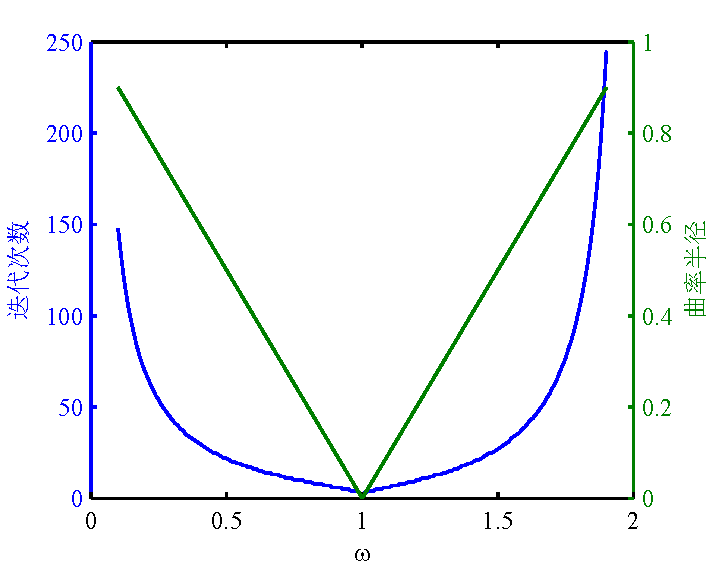图1 问题3的SOR迭代法计算结果(计算精度为1E-4)

4. 共轭梯度法
共轭梯度法与之前的迭代法不同，它属于不定常迭代法，用于求解对称正对线性方程组，其本质上是一种变分方法。  共轭梯度法的迭代方程为

x(k+1)=x(k)+αkp(k)

{x^{\left( {k + 1} \right)}} = {x^{\left( k \right)}} + {\alpha _k}{p^{\left( k \right)}}
其中

αk=∥∥r(k)∥∥22/(Ap(k),p(k))
${\alpha _k}=\left\| r^\left( k \right)\right\|_2^2 / \left( Ap^{(k)},p^{(k)}\right)$,

p(k)
${p^{\left( k \right)}}$为第

k
$k$次迭代过程中的搜索方向，它与梯度方向 r(k)${r^{\left( k \right)}}$ 相关，即

p(k+1)=r(k+1)+βkp(k)

{p^{\left( {k + 1} \right)}} = {r^{\left( {k + 1} \right)}} + {\beta _k}{p^{\left( k \right)}}
其中

r(k+1)=r(k)−αAp(k)

{r^{\left( {k + 1} \right)}} = {r^{\left( k \right)}} - \alpha A{p^{\left( k \right)}}

βk=∥∥r(k+1)∥∥22/∥∥r(k)∥∥22

{\beta _k} = \left\| r^\left( k+1 \right)\right\|_2^2/\left\| r^\left( k \right)\right\|_2^2
当

∥βk∥<TOL
$\left\| {{\beta _k}} \right\| < TOL$时，则迭代结束，其中

TOL
$TOL$为允许误差。
对于初始解，共轭迭代法要求其为最速下降方向，这可以保证所有的迭代过程中的

x(k)
${x^{\left( k \right)}}$都是共轭的，且具有较快地收敛速度。
针对问题(1)(2)(3)中的算例，共轭迭代法首先要求对系数矩阵作对角化处理，即

b=ATb,A=ATA

b = {A^{\rm{T}}}b,A = {A^{\rm{T}}}A
其计算结果如表3所示。

表3 问题1,2,3的共轭迭代法计算结果
问题序号迭代次数计算误差允许误差127.9422E-52118.8953E-51E-4341.9327E-5
由表3所得，共轭迭代法在问题(1)(2)(3)中的线性方程组求解上具有较好的收敛效果，尤其对问题(2)中的大型稀疏矩阵上，其收敛效果明显高于Gauss-Seidel迭代法。
四、实验结论
线性方程组的直接求法，Gauss消去法和LU分解法在维度较小的非病态问题上，具有良好的效果。这两种算法理论上的复杂度为

O(n3)
$O\left( {{n^3}} \right)$，使其能有效地求出低维非病态线性方程组的精确解，但在髙维度或病态的线性方程组问题上出现无法在有限时间内收敛或无法求解的情况。  线性方程组的迭代求法，Jacobi迭代法、Gauss-Seidel迭代法、超松弛(SOR)迭代法和共轭梯度法针对大型稀疏矩阵（如问题(2)的84阶线性方程组）表现出不同的效果。Jacobi迭代法的不收敛、Gauss-Seidel迭代法的极大的迭代次数以及共轭梯度法较优的计算结果，表明了这些迭代法不同的计算特性。SOR迭代法的松弛因子

ω
$\omega$ 对算法的收敛性影响较大，同时，在问题(3)的分析与求解过程中，我们发现谱半径与SOR迭代法呈正相关特性。  基于以上实验结果，我们得到以下结论：  1. 直接求法在一般问题，维数较小下(系数矩阵的秩小于100)，非常实用。  2. 迭代法的收敛性在不同问题反映出的现象差异很大。  3. 采用Jacobi迭代法、Gauss-Seidel迭代法求解大型稀疏矩阵，收敛性难以保证，而共轭迭代法收敛极好。  4. 超松弛迭代法的收敛性受松弛因子影响，并可能存在最优的松弛因子，使其收敛速度最快。
五、实验程序
所有程序都在MATLAB(R2012b)平台上实现。
1. Gauss 消去法
源代码
function [rankA, rankB, N, X] = CNumbericGauss(A, b)
% 调用格式: [rankA, rankB, N, X] = CNumbericGauss(A, b)
%
% 作者: 王瑞
% 时间: 2015.10.27 17:12 - 20.12
% 版本: Version 1.0
%
% 任务: 高斯消去法求解线性方程组的解 Ax = b, 换主元的高斯消去法, 取最邻近非 0 首项
%
% 输入: A = 系数矩阵, 方阵
%       b = 常系数向量, 行向量
%
% 输出: rankA = 系数矩阵 A 的秩
%       rankB = 增广矩阵 B 的秩, 其中 B = [A|b]
%       N = 方程组未知量个数
%       X = 方程组的解向量
%

B = [A b];
rankA = rank(A);
rankB = rank(B);
N = length(b);
X = zeros(size(b));
if rankA ~= rankB
disp('None: 矩阵 A 的秩不等于 [A b] 的秩， 无解！');
return ;
end
if rankA < N
disp(['Waring: 矩阵的秩小于' num2str(N) '，存在无穷多解。']);
return;
end
if N == 1
disp('别闹，用手算的。');
return;
end
%% Gauss 消去法
if rankA == N
disp(['Good: 矩阵的秩为' num2str(N) '，存在唯一解。']);
for i = 1 : N
if abs(B(i, i)) <= eps
B(i, i) = 0;
[flag, j] = max(abs(B(i:N, i)));
j = j + i -1;
temp = B(i, :);
B(i, :) = B(j, :);
B(j, :) = temp;

if abs(flag) <= eps        % 无非 0 首项判定
disp('这里出现了一个 Error，首项极小！');
return;
end
end
B(i, :) = B(i, :)/B(i, i);
for j = i+1 : N         % Gauss 消去
B(j, :) = B(j, :) - B(j, i)*B(i, :);
end
end
for i = N : -1 : 1          % Gauss 回代求解
if i == N
X(i) = B(i, N+1)/B(i, N);
else
X(i) = (B(i, N+1) - B(i, i+1:N)*X(i+1:N)) / B(i, i);
end
end
end
end
2. LU分解法
源代码
function [rankA, rankB, N, X] = CNumbericLU(A, b)
% 调用格式: [rankA, rankB, N, X] = CNumbericLU(A, b)
%
% 作者: 王瑞
% 时间: 2015.10.27 20:14 - 21:37
% 版本: Version 1.0
%
% 任务: 选主元的三角分解法求解线性方程组的解 Ax = b, LU 法
%
% 输入: A = 系数矩阵, 方阵
%       b = 常系数向量, 行向量
%
% 输出: rankA = 系数矩阵 A 的秩
%       rankB = 增广矩阵 B 的秩, 其中 B = [A|b]
%       N = 方程组未知量个数
%       X = 方程组的解向量
%
B = [A b];
rankA = rank(A);
rankB = rank(B);
N = length(b);
X = zeros(size(b));
if rankA ~= rankB
disp('None: 矩阵 A 的秩不等于 [A b] 的秩， 无解！');
return ;
elseif rankA ~= N
disp(['Waring: 矩阵的秩小于' num2str(N) '，存在无穷多解。']);
return;
end
if rankA == 1
disp('别闹，用手算的。');
return;
end
disp(['Good: 矩阵的秩为' num2str(N) '，存在唯一解。']);
%% 求 L U P [核心]
[L, U, P] = lu(A);
b = P*b;
%% 求 y
y = zeros(size(b));
for i = 1 : N
if i == 1
y(1) = b(1);
else
y(i) = b(i) - L(i, 1:i-1)*y(1:i-1);
end
end
%% 求 X
for i = N : -1 : 1
if i == N
X(i) = y(i) / U(i, i);
else
X(i) = (y(i) - U(i, i+1:N)*X(i+1:N)) / U(i, i);
end
end
end

3. Jacobi迭代法
源代码
function [rankA, rankB, N, X, ite, tol] = CNumbericJacobiIteration(A, b, TOL, ITE, initX)
% 调用格式: [rankA, rankB, N, X, ite, tol] = CNumbericJacobiIteration(A, b, initX, TOL, ITE, initX)
%           [rankA, rankB, N, X, ite, tol] = CNumbericJacobiIteration(A, b, initX, TOL, ITE)
%
% 作者: 王瑞
% 时间: 2015.10.27 21:40; 2015.10.28 13:37 - 15:00
% 版本: Version 1.0
%
% 任务: Jacobi迭代法求解线性方程组的解 Ax = b
%       构建 x(k+1) = Bx(k) + f
%       B = E - D^-1*A = D^-1*(L+U), f = D^-1*b;
%
% 输入: A = 系数矩阵, 方阵
%       b = 常系数向量, 行向量
%       initX = 初始解
%       ITE = 迭代次数上限
%       TOL = 解的精度(范数)
%
% 输出: rankA = 系数矩阵 A 的秩
%       rankB = 增广矩阵 B 的秩, 其中 B = [A|b]
%       N = 方程组未知量个数
%       X = 方程组的解向量
%       ite = 求解的迭代次数
%       tol = 实际误差
%
if nargin == 5
X = initX;
elseif nargin == 4
X = zeros(size(b));
end

B = [A, b];
rankA = rank(A);
rankB = rank(B);
N = length(b);
ite = 0;
tol = inf;
if rankA ~= rankB
disp('None: 矩阵 A 的秩不等于 [A b] 的秩， 无解！');
return ;
elseif rankA ~= N
disp(['Waring: 矩阵的秩小于' num2str(N) '[' num2str(rankA) ']''，存在无穷多解。']);
end
if rankA == 1
disp('别闹，用手算的。');
return;
end
if rankA == N
disp(['Good: 矩阵的秩为' num2str(N) '，存在唯一解。']);
end
%% B0, f
D = diag(A);
L = (-1) * tril(A, -1);
U = (-1) * triu(A, 1);
invD = diag(1./D);
B0 = invD*(L+U);
f = invD*b;
R = max(abs(eig(B0)));              % 谱半径，收敛判定
if R >= 1
disp(['Error: 谱半径 R = ' num2str(R) '，大于等于 1，算法不收敛。']);
return;
end
%% Jacobi 迭代
while ite < ITE
ite = ite + 1;
X = B0*X + f;
tol = norm(b - A*X) / norm(b);
if tol < TOL
disp('Excatly: 求得解。');
break;
end
end
if ite > ITE
disp(['Message: 在 ' num2str(ITE) ' 次迭代过程中，无法求得解，'...
'建议增大总的迭代次数 或者 分析算法的收敛性。']);
end
end

4. Gauss-Seidel迭代法
源代码
function [rankA, rankB, N, X, ite, tol] = CNumbericGaussSeidelIteration(A, b, TOL, ITE, initX)
% 调用格式: [rankA, rankB, N, X, ite, tol] = CNumbericGaussSeidelIteration(A, b, TOL, ITE, initX)
%           [rankA, rankB, N, X, ite, tol] = CNumbericGaussSeidelIteration(A, b, TOL, ITE)
%
% 作者: 王瑞
% 时间: 2015.10.28 14:15 - 15:00
% 版本: Version 1.0
%
% 任务: Gauss-Seidel迭代法求解线性方程组的解 Ax = b
%       构建 x(k+1) = Gx(k) + f
%       G = (D - L)^-1*U, f = (D - L)^-1*b
%
% 输入: A = 系数矩阵, 方阵
%       b = 常系数向量, 行向量
%       initX = 初始解
%       ITE = 迭代次数上限
%       TOL = 解的精度(范数)
%
% 输出: rankA = 系数矩阵 A 的秩
%       rankB = 增广矩阵 B 的秩, 其中 B = [A|b]
%       N = 方程组未知量个数
%       X = 方程组的解向量
%       ite = 求解的迭代次数
%       tol = 实际误差
%
if nargin == 5
X = initX;
elseif nargin == 4
X = zeros(size(b));
end

B = [A, b];
rankA = rank(A);
rankB = rank(B);
N = length(b);
ite = 0;
tol = inf;
if rankA ~= rankB
disp('None: 矩阵 A 的秩不等于 [A b] 的秩， 无解！');
return ;
elseif rankA ~= N
disp(['Waring: 矩阵的秩小于' num2str(N) '[' num2str(rankA) ']''，存在无穷多解。']);
end
if rankA == 1
disp('别闹，用手算的。');
return;
end
if rankA == N
disp(['Good: 矩阵的秩为' num2str(N) '，存在唯一解。']);
end
%% G, f
D = diag(diag(A));
L = (-1) * tril(A, -1);
U = (-1) * triu(A, 1);
invDL = eye(length(b))/(D - L);
G = invDL*U;
f = invDL*b;
R = max(abs(eig(G)));       % 谱半径，收敛判定
if R >= 1
disp(['Error: 谱半径 R = ' num2str(R) '，大于等于 1，算法不收敛。']);
return;
end
%% Gauss-Seidel 迭代
while ite < ITE
ite = ite + 1;
Xk = X;
X = G*Xk + f;
tol = norm(b - A*X) / norm(b);
if tol < TOL
disp('Excatly: 求得解。');
break;
end
end
if ite > ITE
disp(['Message: 在 ' num2str(ITE) ' 次迭代过程中，无法求得解，'...
'建议增大总的迭代次数 或者 分析算法的收敛性。']);
end
end

5. 超松弛(SOR)迭代法
源代码
function [rankA, rankB, N, X, ite, tol] = CNumbericSORIteration(A, b, Omega, TOL, ITE, initX)
% 调用格式: [rankA, rankB, N, X, ite, tol] = CNumbericSORIteration(A, b, Omega, TOL, ITE, initX)
%           [rankA, rankB, N, X, ite, tol] = CNumbericSORIteration(A, b, Omega, TOL, ITE)
%
% 作者: 王瑞
% 时间: 2015.10.28 16:00 - 16:38
% 版本: Version 1.0
%
% 任务: 超松弛(SOR)迭代法求解线性方程组的解 Ax = b
%       构建 x(k+1) = Gx(k) + f
%       G = (D-Omege*L)^-1*[(1-Omega)*D+Omega*U],
%       f = Omega*(D-Omega*L)^-1*b
%
% 输入: A = 系数矩阵, 方阵
%       b = 常系数向量, 行向量
%       initX = 初始解
%       Omege = 松弛因子 (0, 2)
%       ITE = 迭代次数上限
%       TOL = 解的精度(范数)
%
% 输出: rankA = 系数矩阵 A 的秩
%       rankB = 增广矩阵 B 的秩, 其中 B = [A|b]
%       N = 方程组未知量个数
%       X = 方程组的解向量
%       ite = 求解的迭代次数
%       tol = 实际误差
%
if nargin == 5
X = zeros(size(b));
elseif nargin == 6
X = initX;
end

B = [A, b];
rankA = rank(A);
rankB = rank(B);
N = length(b);
ite = 0;
tol = inf;
if rankA ~= rankB
disp('None: 矩阵 A 的秩不等于 [A b] 的秩， 无解！');
return ;
elseif rankA ~= N
disp(['Waring: 矩阵的秩小于' num2str(N) '[' num2str(rankA) ']''，存在无穷多解。']);
end
if rankA == 1
disp('别闹，用手算的。');
return;
end
if rankA == N
disp(['Good: 矩阵的秩为' num2str(N) '，存在唯一解。']);
end
%% D, L, U
D = diag(diag(A));
L = (-1) * tril(A, -1);
U = (-1) * triu(A, 1);
%% G, f
G = (D - Omega*L)\((1-Omega)*D + Omega*U);
f = (D - Omega*L)\(Omega*b);
R = max(abs(eig(G)));       % 谱半径，收敛判定
if R >= 1
disp(['Error: 谱半径 R = ' num2str(R) '，大于等于 1，算法不收敛。']);
return;
end
disp(['R = ' num2str(R)]);
%% SOR 迭代
while ite < ITE
ite = ite + 1;
X = G*X + f;
tol = norm(b - A*X) / norm(b);
if tol < TOL
disp('Excatly: 求得解。');
break;
end
end
if ite > ITE
disp(['Message: 在 ' num2str(ITE) ' 次迭代过程中，无法求得解，'...
'建议增大总的迭代次数 或者 分析算法的收敛性。']);
end
end

6. 共轭梯度法
源代码
function [rankA, rankB, N, X, ite, tol] = CNumbericCGMIteration(A, b, TOL, ITE, initX)
% 调用格式: [rankA, rankB, N, X, ite, tol] = CNumbericCGMIteration(A, b, TOL, ITE, initX)
%           [rankA, rankB, N, X, ite, tol] = CNumbericCGMIteration(A, b, TOL, ITE)
%
% 作者: 王瑞
% 时间: 2015.10.28 16:54 - 19:21
% 版本: Version 1.0
%
% 任务: 共轭梯度法(Conjugate Gradient Method, CGM)迭代法求解线性方程组的解 Ax = b
%       适用于系数矩阵为对称阵的线性方程组(函数内包含矩阵对称化 b = A'*b, A = A'*A)
%       构建 x(k+1) = x(k) + alpha(k)*p(k)
%       等同 MATLAB 内置函数 cgs
%
% 输入: A = 系数矩阵, 方阵
%       b = 常系数向量, 行向量
%       ITE = 迭代次数上限
%       TOL = 解的精度(范数)
%       initX = 初始解
%
% 输出: rankA = 系数矩阵 A 的秩
%       rankB = 增广矩阵 B 的秩, 其中 B = [A|b]
%       N = 方程组未知量个数
%       X = 方程组的解向量
%       ite = 求解的迭代次数
%       tol = 实际误差
%
if nargin == 5
X = initX;
elseif nargin == 4
X = zeros(size(b));
end
%% 解的判定
B = [A, b];
rankA = rank(A);
rankB = rank(B);
N = length(b);
ite = 0;
tol = inf;
if rankA ~= rankB
disp('None: 矩阵 A 的秩不等于 [A b] 的秩， 无解！');
return ;
elseif rankA ~= N
disp(['Waring: 矩阵的秩小于' num2str(N) '[' num2str(rankA) ']''，存在无穷多解。']);
end
if rankA == 1
disp('别闹，用手算的。');
return;
end
if rankA == N
disp(['Good: 矩阵的秩为' num2str(N) '，存在唯一解。']);
end
% 系数矩阵对称，放大 A' 倍
if rank(A-A') ~= 0
b = A'*b;
A = A'*A;
end
% GCM 迭代
r = b - A*X;
while ite < ITE
err = r'*r;
ite = ite + 1;
if ite == 1
p = r;
else
beta = err / errold;
p = r + beta*p;
end
Ap = A*p;
alpha = err / ((Ap)'*p);
X = X + alpha*p;
r = r - alpha*Ap;
errold = err;
tol = norm(r) / norm(b);
if tol < TOL
disp('Excatly: 求得解。');
break;
end
end
if ite > ITE
disp(['Message: 在 ' num2str(ITE) ' 次迭代过程中，无法求得解，'...
'建议增大总的迭代次数 或者 分析算法的收敛性。']);
end
end
展开全文线性方程组 共轭梯度法 超松弛迭代 Gauss消去法 迭代法
• 以∞-范数为媒介，容易证明有限上不同范数是等价的。 matlab中求向量范数使用norm(v)，默认2-范数。若想指定p值，传入两个参数norm（vector,p）即可。实例见matlab_norm 矩阵范数 定义如下 ∣∣A∣∣=max⁡x≠0∣...算法 matlab 矩阵 线性代数
• 《偏微分方程数值解法MATLAB源码》由会员分享，可在线阅读，更多相关《偏微分方程数值解法MATLAB源码(27页珍藏版)》请在人人文库网上搜索。1、源码【更新完毕】偏微分方程数值解法的MATLAB原创 说明：由于偏微分的...
• 输入数值n，表示块nxn的区域，其中数值1表示信号强，0表示信号弱，例如： ...思路：将整个nxn数组建立成个二图，利用广度优先搜索算法进行搜索，直到命中右小角元素。 代码如下： #include <iostre...C++编程 面试题
• 给定个包含了一些 0 和 1 的非空二数组 grid 。 个 岛屿 是由一些相邻的 1 (代表土地) 构成的组合，这里的「相邻」要求两个 1 必须在水平或者竖直方向上相邻。你可以假设 grid 的四个边缘都被 0（代表水）包围...
• 《双曲方程基于matlab的数值解法》由会员分享，可在线阅读，更多相关《双曲方程基于matlab的数值解法(9页珍藏版)》请在人人文库网上搜索。1、双曲型方程基于MATLAB的数值解法(数学1201,陈晓云,)：一阶双曲型微分...
• 74.搜索矩阵 描述 编写个高效的算法来判断 m x n 矩阵中，是否存在个目标值。该矩阵具有如下特性： 每行中的整数从左到右按升序排列。 每行的第个整数大于前行的最后个整数。 示例 示例 1: 输入: ...
• 240. 搜索矩阵 II 编写个高效的算法来搜索 m x n 矩阵 matrix 中的个目标值 target。该矩阵具有以下特性： 1.每行的元素从左到右升序排列。 2. 每列的元素从上到下升序排列。 示例: 现有矩阵 matrix 如下： ...二分查找
• 74. 搜索矩阵 编写个高效的算法来判断m x n矩阵中，是否存在个目标值。该矩阵具有如下特性： 每行中的整数从左到右按升序排列。 每行的第个整数大于前行的最后个整数。 示例 1： 输入：matrix = ...
• 给定不同面额的硬币 coins 和个总金额 amount。编写个函数来计算可以凑成总金额所需的最少的硬币个数。如果没有任何种硬币组合能组成总金额，返回 -1。 示例 1: 输入: coins = [1, 2, 5], amount = 11 输出: 3...java 算法 动态规划 数据结构
• 在一个二维数组中（每个一维数组的长度相同），每一行都按照从左到右递增的顺序排序，每一列都按照从上到下递增的顺序排序。请完成一个函数，输入这样的一个二维数组和一个整数，判断数组中是否含有该整数。 思路 本...
• 文章目录1.遗传算法1.1算法概述1.2案例讲解1.3改进2.蚁群算法2.1算法概述2.2案例分析 1.遗传算法 1.1算法概述 遗传算法( genetic algorithm,...在遗传算法中,染色体对应的是数据或数组,通常是由一维的串结构数据来表示算法 人工智能 机器学习
• 华电北风吹 最后修改日期 2015/11/7无约束极值问题可以表述为 ...另类是迭代过程中只用到函数值的直接法。常见的解析法有梯度下降法，共轭梯度法，变尺度法。常见的直接法有步长加速法。、梯度
• N皇后问题是个经典的问题，在个N*N的棋盘上放置N个皇后，每行个并使其不能互相攻击（同一行、同一列、同一斜线上的皇后都会自动攻击）。 n皇后问题不算是陈词滥调，也是老生常谈了，作为回溯的经典案例，有...
• 本文介绍了ZOJ1008，Gnome Tetravex游戏的种解题思路算法 递归 ACM C++
• 幅以二整数数组表示的图画，每个整数表示该图画的像素值大小，数值在 0 到 65535 之间。 给你个坐标 (sr, sc) 表示图像渲染开始的像素值（行 ，列）和个新的颜色值 newColor，让你重新上色这幅图像。 ...leetcode
• 计算机辅助数值分析(Computer-aided numerical analysis，CAA) 是以电子计算机为主要工具的种分析与设计方法。它是以计算技术、应用数学与模拟理论为基础发展起来的一门新兴学科，目前已成为计算机应用领域中个...
• 二分搜索与三分搜索的应用： 二分和三分都利用了分治的思想,都是通过不断缩小查找的范围,把问题分解为更小的子问题直到找到...、二分搜索 (1)、应用二分最常见或者说最基础的的就是从有序序列中查找某个值：查找等ACM学习
• 宽度优先搜索之习题分析、宽度优先搜索的概念二、小岛问题（）、题目需求（二）、解法（三）、代码分析三、单词梯（）、题目需求（二）、解法（三）、代码分析 、宽度优先搜索的概念 ​ 广度优先搜索（也称...字符串 队列 算法 bfs
• 深度优先搜索的核心思想就是条道走到黑，其实就和二叉树的前、中、后序遍历一样，都会先一直沿着个方向走，当走不通了再往回找其他的路。 //模板 DFS(当前这一步的处理逻辑) { 1. 判断边界，是否已经条道走...算法 BFS DFS
• 通过（暴力法、深度优先搜索、直线方程）3种方法，判断两壶水拼凑水量的问题...直线方程
• 在一个二维数组中（每个一维数组的长度相同），每一行都按照从左到右递增的顺序排序，每一列都按照从上到下递增的顺序排序。请完成一个函数，输入这样的一个二维数组和一个整数，判断数组中是否含有该整数。 利用...
• 使用导数的最优化算法中，拟牛顿法是目前为止最为行之有效的一种算法，具有收敛速度快、算法稳定性强、编写程序容易等优点。在现今的大型计算程序中有着广泛的应用。本文试图介绍拟牛顿法的...一维情况下，也即令函数为
• 记录leetcode的一些解法1. Two Sum2. Add Two Numbers 1. Two Sum Given an array of integers, return indices of the two numbers such that they add up to a specific target. You may assume that each ...leetcode
• 上篇文章讲动态规划获得了80k浏览，这次的二分也值得你们看，这个系列是特别用心准备出书的哦
• 入门： 1. 反转字符串 描述 写出个程序，接受个字符串，然后输出该字符串反转后的字符串。（字符串长度不超过1000） 示例1测试工程师
• ## 算法题总结+解法

千次阅读 2014-12-10 13:01:42
原题转载自：... 解法为原创，红色字体标出。 ...输入棵二元查找树，将该二元查找树转换成个排序的双向链表。 要求不能创建任何新的结点，只调整指针的指向。  10  / \  6 14算法 数据结构...